# INDEX & MATCH in Excel - a better alternative to VLOOKUP

This tutorial demonstrates the key strengths of Excel's INDEX / MATCH function that make it superior to VLOOKUP. You will find a number of formula examples that will help you easily cope with many complex tasks when VLOOKUP fails.

In a couple of recent articles I made a good effort to explain the basics of Excel VLOOKUP function to beginners and provide more complex VLOOKUP formula examples to power users. And now, I will try if not to talk you out of using VLOOKUP, then at least show you an alternative way to do a vertical lookup in Excel.

"What do I need that for?" you can ask me. Because VLOOKUP is not the only lookup formula available in Excel, and its numerous limitations might prevent you from getting the desired result in many situations. On the other hand, Excel's INDEX MATCH is more flexible and has certain features that make it superior to VLOOKUP in many respects.## Excel's INDEX and MATCH functions - the basics

Since the aim of this tutorial is to demonstrate an alternative way to do a vlookup in Excel by using a combination of INDEX and MATCH functions, we won't dwell much on their syntax and uses. We will cover only the minimum necessary for understanding the general idea and then take an in-depth look at formula examples that reveal all the advantages of using Index / Match instead of Vlookup.

#### The INDEX function's syntax and usage

The Excel INDEX function returns a value in an array based on the row and column numbers you specify. The syntax of the INDEX function is straightforward:

INDEX(array, row_num, [column_num])

Here is a very simple explanation of each parameter:

• array - a range of cells that you want to return a value from.
• row_num - the row number in array from which you want to return a value. If omitted, the column_num is required.
• column_num - the column number in array from which you want to return a value. If omitted, row_num is required.

And here is the simplest example of the INDEX formula:

`=INDEX(A1:C10,2,3)`

The formula searches in cells A1 through C10 and returns a value of the cell in the 2nd row and the 3rd column, i.e. cell C2.

Very easy, right? However, when working with real data you would hardly ever know which row and column you want, that is why you need the help of the MATCH function.

#### The MATCH function's syntax and usage

The Excel MATCH function searches for a lookup value in a range of cells, and returns the relative position of that value in the range.

For example, if the range B1:B3 contains the values "New-York", "Paris", "London", then the formula `=MATCH("London",B1:B3,0)` returns the number 3, because "London" is the third entry in the range.

The syntax of the MATCH function is as follows:

MATCH(lookup_value, lookup_array, [match_type])
• lookup_value - the number or text value you are looking for.
• lookup_array - a range of cells being searched.
• match_type - specifies whether you want to return an exact match or the nearest match:
• 1 or omitted - finds the largest value that is less than or equal to the lookup value. The values in the lookup array must be sorted in ascending order.
• 0 - finds the first value that is exactly equal to the lookup value. In the INDEX / MATCH combination, you almost always need the exact match, so the third argument of your MATCH function is "0".
• -1 - finds the smallest value that is greater than or equal to lookup_value. The values in the lookup array must be sorted in descending order.

At first sight, the usefulness of the MATCH function may seem questionable. Who cares about the position of a value in a range? What we do want to know is the value itself.

Let me remind you that the relative position of the lookup value (i.e. a row or/and column number) is exactly what you need to supply to the row_num or/and column_num argument of the INDEX function. As you remember, the INDEX function can return the value at the juncture of a given row and column, but it cannot determine which exactly row and column you want.

## How to use INDEX MATCH function in Excel

Now that you know the basics of these two functions, I believe it has already started making sense how Excel's MATCH and INDEX work together.

The MATCH function determines the relative position of the lookup value in the specified range of cells. From there, the INDEX function takes that number, or numbers, and returns a value in the corresponding cell.

Still having difficulties to figure it out? Think about Excel INDEX / MATCH in this way:

INDEX (column to return a value from, MATCH (lookup value, column to look up against, 0))

I believe it's even easier to understand from an example. Suppose you have a list of national capitals like this: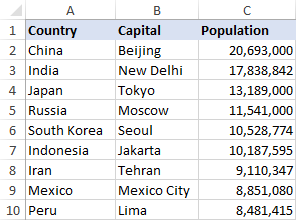Let's find the population of some capital, say the capital of Japan, using the following Index Match formula:

`=INDEX(\$D\$2:\$D\$10,MATCH("Japan",\$B\$2:\$B\$10,0))`

Now, let's analyze what each component of this formula actually does:

• The MATCH function searches for the lookup value "Japan" in the range B2:B10, and returns the number 3, because "Japan" is third in the lookup array.
• The relative position of the lookup value goes directly to the row_num argument of INDEX instructing it to return a value from that row.

So, the above formula turns into a simple INDEX(\$D\$2:\$D\$10,3) that says to search in cells D2 through D10 and return the value of the 3rd cell in that range, i.e. cell D4 because we start counting from the second row.

And here's the result you get in Excel: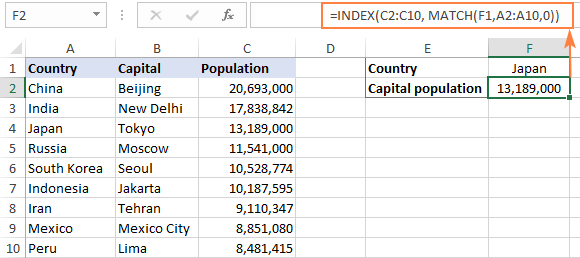Important! The number of rows in the array argument of INDEX should match the number of rows in the lookup_array argument of MATCH, otherwise, the formula will return an incorrect result.

Wait, wait... why don't we simply use the following Vlookup formula? What's the point in wasting time trying to figure out the arcane twists of Excel's MATCH / INDEX?

`=VLOOKUP("Japan",\$B\$2:\$D\$2,3)`

In this case, no point at all : ) This simple example is for demonstration purposes only, so that you get a feel of how the Index and Match functions work together. Other examples that follow below will show you the real power of the INDEX MATCH liaison that easily copes with many complex scenarios when VLOOKUP stumbles.

## Why Excel's INDEX MATCH is better than VLOOKUP

When deciding which formula to use for vertical lookups, the majority of Excel gurus agree that INDEX / MATCH is far better than VLOOKUP. However, many Excel users still resort to utilizing VLOOKUP because it's a simpler function. This happens because very few people fully understand all the benefits of switching from Vlookup to Index Match, and without such understanding no one is willing to invest their time to learn a more complex formula.

Below, I will try to point out the key advantages of using MATCH / INDEX in Excel, and then you decide whether you'd rather stick with Vlookup or switch to Index Match.

#### 4 top benefits of using MATCH INDEX in Excel

1. Right to left lookup. As any educated user knows, Excel VLOOKUP cannot look to its left, meaning that your lookup value should always reside in the left-most column of the lookup range. INDEX MATCH can do a left lookup with ease! The following example demonstrates this feature in action - How to vlookup a value to the left in Excel.
2. Insert or delete columns safely. VLOOKUP formulas get broken or return incorrect results when a new column is deleted from or added to a lookup table. With VLOOKUP, any inserted or deleted column changes the results returned by your formulas because the VLOOKUP function's syntax requires specifying the entire table array and a certain number indicating which column you want to pull the data from.

For example, if you have a table A1:C10 and want to return a value from column B, you will put "2" in the third parameter (col_index_num) of a VLOOKUP formula, say `=VLOOKUP("lookup value", A1:C10, 2)`. If at a later point, you insert a new column between A and B, you will have to change "2" to "3" in your formula, otherwise it would return a value from the newly inserted column.

With INDEX MATCH, you can delete or insert new columns in your lookup table without distorting the results since you specify directly the column containing the value you want to get. And this is a really great benefit, especially when working with large datasets, since you are able to insert and remove columns without worrying about updating every associated vlookup formula.

3. No limit for a lookup value's size. When using the VLOOKUP function, remember that the total length of your lookup criteria should not exceed 255 characters, otherwise you will end up having the #VALUE! error. So, if your dataset contains long strings, INDEX MATCH is the only working solution.

Suppose, you are using the following VLOOKUP formula that searches through cells B5 to D10 for the value in cell A2:

`=VLOOKUP(A2,B5:D10,3,FALSE)`

The formula won't work if the lookup value in cell A2 exceeds 255 symbols. Instead, you shall use the analogous INDEX / MATCH function:

`=INDEX(D5:D10, MATCH(TRUE, INDEX(B5:B10=A2, 0), 0))`

4. Higher processing speed. If your tables are relatively small, there will hardly be any significant difference in Excel's performance. But if your worksheets contain hundreds or thousands of rows, and consequently hundreds or thousands of formulas, MATCH INDEX will work much faster than VLOOKUP because Excel will have to process only the lookup and return columns rather than the entire table array.

VLOOKUP's impact on Excel's performance may be especially noticeable if your workbook contains complex array formulas like VLOOKUP and SUM. The point is that checking each value in the array requires a separate call of the VLOOKUP function. So, the more values your array contains and the more array formulas you have in a workbook, the slower Excel performs.

On the other hand, with INDEX MATCH, Excel has to consider only the lookup and return columns, as the result it processes such formulas much faster.

## Excel INDEX & MATCH - formula examples

Now that you know the reasons to learn the MATCH INDEX function, let's get to the most interesting part and see how you can apply the theoretical knowledge in practice.

### How to look up from right to left with INDEX & MATCH

As stated in any VLOOKUP tutorial, this Excel function cannot look at its left. So, unless your lookup column is the left-most column in the lookup range, there's no chance that a vlookup formula will return the result you want.

Excel's INDEX MATCH function is more flexible and does not really care where the return column resides. As an example, we will use the table listing national capitals by population again. This time, let's write an INDEX MATCH formula that finds how the Russian capital, Moscow, ranks in terms of population.

As you can see in the screenshot below, the following formula has not problem with performing a left vlookup:

`=INDEX(\$A\$2:\$A\$10,MATCH("Russia",\$B\$2:\$B\$10,0))`

Naturally, you can replace the "hard-coded" lookup value with a cell reference:

`=INDEX(\$A\$2:\$A\$10,MATCH(F2,\$B\$2:\$B\$10,0))`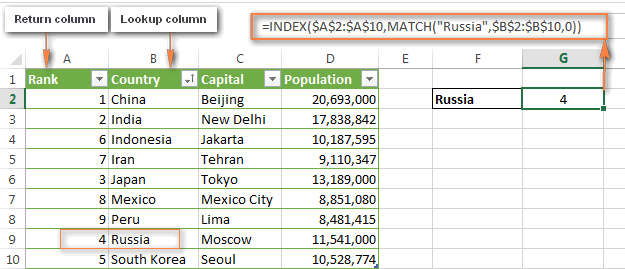By now, you should not have any difficulties to understand how the formula works:

• First, you write a simple MATCH formula that finds the position of Russia: `=MATCH("Russia",\$B\$2:\$B\$10,0))`
• Then, you determine the array parameter for your Index function, which is column A in our case (A2:A10).
• Finally, you assemble the two parts together and get this formula: `=INDEX(\$A\$2:\$A\$10,MATCH("Russia",\$B\$2:\$B\$10,0))`
Tip. It's a good idea to always use absolute cell references in INDEX and MATCH formulas so that your lookup ranges won't get distorted when you copy the formula to other cells.

### Calculations with INDEX MATCH in Excel (AVERAGE, MAX, MIN)

You can nest other Excel functions within the MATCH INDEX formula, say, to find the minimum or maximum value, or the value closest to the average in the range. Here are a few formula examples for the table used in the previous sample:

 Function Formula example Description Returned result Min =INDEX(\$C\$2:\$C\$10, MATCH(MIN(\$D\$2:I\$10), \$D\$2:D\$10, 0)) Finds the min value in column D and returns a value from column C in the same row. Beijing Max =INDEX(\$C\$2:\$C\$10, MATCH(MAX(\$D\$2:I\$10), \$D\$2:D\$10, 0)) Finds the max value in column D and returns a value from column C in the same row. Lima Average =INDEX(\$C\$2:\$C\$10, MATCH(AVERAGE(\$D\$2:D\$10), \$D\$2:D\$10, 1)) Calculates the average in range D2:D10, finds the value closest to the average, and returns a corresponding value from column C. Moscow

#### Things to keep in mind when using AVERAGE with INDEX / MATCH

When using the AVERAGE function in combination with INDEX MATCH, most often you would enter either "1" or "-1" in the third argument (match_type) of the MATCH function, unless you are certain that your lookup array contains a value exactly equal to the average. If latter, you can enter "0" for exact match.

If you set 1, the values in the lookup column must be sorted in ascending order, and the formula will return the largest value that is less than or equal to the average value.

If you set -1, the values in the lookup column must be sorted in descending order, and the smallest value that is greater than or equal to the average value will be returned.

In our example, values in column D are sorted in ascending order, so we use "1" as the match type, and our Average + Index Match formula returns "Moscow" since its population (11,5410,00) is the closest "less than" match to the average number (12,269,006).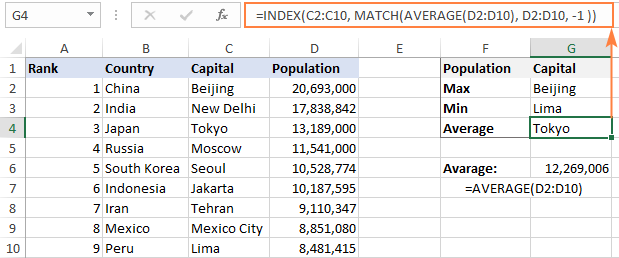If you are curious to know analogues VLOOKUP formulas, here you go: Calculations with VLOOKUP (AVERAGE, MAX, MIN).

### How to use INDEX MATCH to search by row and column values

This formula is an equivalent of the two-dimensional VLOOKUP that lets you find the value at the intersection of a certain row and column.

In this example, your Excel INDEX MATCH function is going to be very similar to other formulas we've already discussed in this tutorial, with the only difference. Guess what?

As you remember, the syntax of the INDEX function allows for both: `INDEX(array, row_num, [column_num])`. And I congratulate those of you who have guessed right : )

To begin with, let's create the general formula to perform a matrix lookup. We just take the INDEX / MATCH formula you already know and add one more MATCH function to it, which will return the column number:

=INDEX (lookup table, MATCH (vertical lookup value, column to look up against, 0), MATCH (horizontal lookup value, row to lookup against, 0))

Please pay attention that you have to specify the entire table in the array argument of the INDEX function in case of two-way lookup.

And now, let us apply this formula template in practice. Below, you see a list of the most populated counties in the world. Suppose, you want to know the number of population in the USA in the year 2015: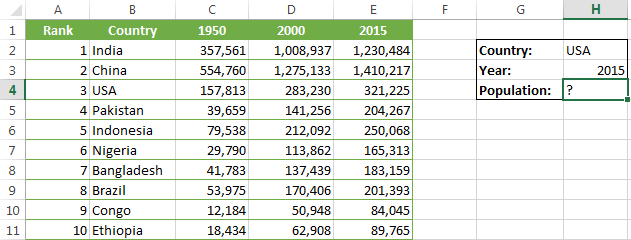Okay, let's start on the formula. Whenever I need to create a complex Excel formula with one or several nested functions, I always write each individual function first.

So, you start by writing two MATCH functions that will return the row and column numbers for your INDEX function.

• Vertical match - you search through column B, more precisely in cells B2 to B11, for the value in cell H2 ("USA"), and the corresponding MATCH function is this: `=MATCH(\$H\$2,\$B\$1:\$B\$11,0)` This MATCH formula returns 4 because "USA" is the 4th item in column B (including the column header).
• Horizontal match - you search for the value in cell H3 ("2015") in row 1, i.e. in cells A1 to E1: `=MATCH(\$H\$3,\$A\$1:\$E\$1,0)` This MATCH formula returns "5" because "2015" is the 5th column.

Now, put the above formulas inside the INDEX function, and voila:

`=INDEX(\$A\$1:\$E\$11, MATCH(\$H\$2,\$B\$1:\$B\$11,0), MATCH(\$H\$3,\$A\$1:\$E\$1,0))`

If you replace the MATCH functions with the returned numbers, the formula is much easier to understand: `=INDEX(\$A\$1:\$E\$11, 4, 5, 0))`

Meaning, it returns a value at the intersection of the 4th row and 5th column in range A1:E11, which is the value in cell E4. Easy? Yep! : )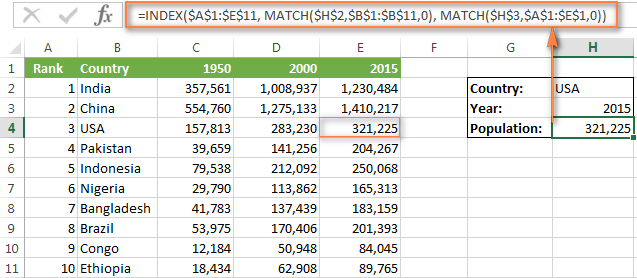### Excel INDEX MATCH with multiple criteria

In the Excel VLOOKUP tutorial, I demonstrated a formula example to do a vlookup with multiple criteria. However, a significant limitation of that approach was the necessity to add a helper column. The good news is that Excel's INDEX MATCH function can also look up values in 2 columns, at that no helper column is requited!

Suppose, you have a list of orders and you want to find the sum based on 2 criteria, "Customer Name" and "Product". A complicating factor is that one customer can buy multiple products and customer names are listed in a random order in the Lookup table: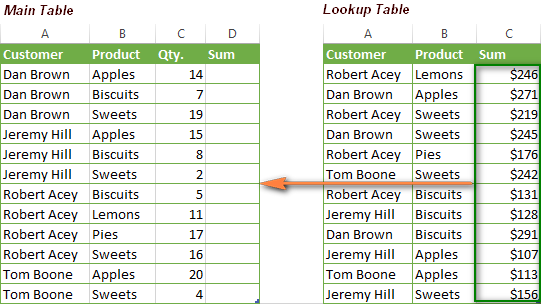The following INDEX MATCH array formula works a treat:

`=INDEX('Lookup table'!\$A\$2:\$C\$13, MATCH(1, (A2='Lookup table'!\$A\$2:\$A\$13) * (B2='Lookup table'!\$B\$2:\$B\$13) ,0), 3)`

This formula is more complex than any other we've discussed today, but powered with the knowledge of Excel MATCH INDEX you already have, I am sure you can cope with it.

The trickiest part is the MATCH function, so let's figure it out first:

`MATCH(1, (A2='Lookup table'!\$A\$2:\$A\$13),0) * (B2='Lookup table'!\$B\$2:\$B\$13), 0)`

What we have here is the following 3 arguments:

• Lookup_value: 1
• Lookup_array: (A2='Lookup table'!\$A\$2:\$A\$13),0) * (B2='Lookup table'!\$B\$2:\$B\$13)
• Match_type: 0

The 1st and 3rd parameters are crystal clear - the function searches for "1", and returns the first found value.

Now, the main question is - why do we look up "1"? To get the answer, let's have a closer look at our lookup array.

What we do here is take the first value (A2) in the "Customer name" column in the main table and check it against all customer names in the lookup table (A2:A13). If the match is found, the equation returns TRUE, otherwise FALSE. Then we do the same for values in columns B ("Products").

To better understand what I am talking about, you can select the arrays in the formula bar, and press the F9 key to see what each of the selected parts evaluates to (please see Evaluating portions of an array formula for more details):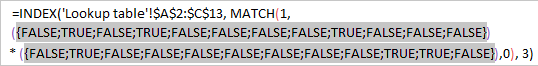As you probably know, in Excel formulas, the logical value of TRUE equates to 1, and FALSE equates to 0. And because the asterisk (*) acts as the AND operator in array formulas, we get "1" only if the match is found in both columns, 0 otherwise. So, the result of this operation is an array of 1's and 0's, where "1" is the value that meets both of the specified conditions. If your lookup table does not have any duplicated rows, there will be just one "1" in the array. And because "1" is our lookup value, the MATCH function returns the relative position of that row as demonstrated in the following screenshot: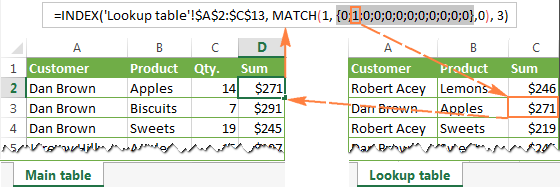Also, please pay attention that you have to use the optional third parameter (column_num) of the INDEX function. This is because you specify the entire table in the first parameter (array), and you need to let the function know which column you want to return a value from. In our case it's column C ("Sum"), so we enter 3 in the third argument of the Index function.

And finally, since we need to check each cell in the array, our INDEX MATCH formula should be an array formula. The most obvious visual indication of an array formula is {curly braces} in which it is encapsulated in the formula bar. Just remember to press Ctrl+Shift+Enter to properly complete the formula after you've finished typing.

If everything is done correctly, you will get a result similar to what you see in the screenshot below: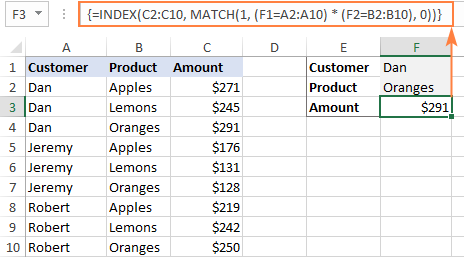### Using INDEX / MATCH with IFERROR in Excel

As you have probably noticed (more than once : ) if you enter an invalid value, i.e. a value that does not exist in the lookup array, the INDEX / MATCH function produces #N/A or #VALUE error message. If you'd rather replace it will something more meaningful, you can wrap your INDEX / MATCH formula in the IFERROR function.

The syntax of the IFERROR function is very simple:

IFERROR(value, value_if_error)

Where the value argument is the value checked for an error (the result of the INDEX MATCH formula in our case); and value_if_error is the value to return if the formula produces an error.

For example, you can enclose the formula from the previous example within the IFERROR function in this way:

`=IFERROR(INDEX(\$A\$1:\$E\$11, MATCH(\$G\$2,\$B\$1:\$B\$11,0), MATCH(\$G\$3,\$A\$1:\$E\$1,0)), "No match is found. Please try again!")`

And now, if someone inputs an invalid entry, the formula will produce the result you see in the screenshot below: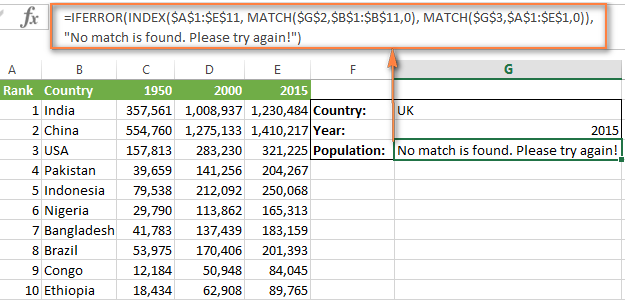If you prefer to have a blank cell when an error is returned, you can just use double quotes ("") in IFERROR's second parameter, like this:

IFERROR(INDEX(array, MATCH(lookup_value, lookup_array, 0), "")

I hope at least one formula described in this tutorial proved to be helpful for you. If you are faced with some other lookup task for which you have not found a solution here, don't hesitate to drop me a comment and we will try to fathom it out.

### 462 Responses to "INDEX & MATCH in Excel - a better alternative to VLOOKUP"

1.J.E says:

Hi,

I'm trying to find something similar to this but I'm having trouble.

I have a list of addresses in Sheet 1, the whole address is in one cell. So for example A2, A3, A4 all contain a different address. Then in B2, B3, B4 etc I want to display a area code, "3" or "8" for example based on the postcode in the cell in column A.

I have a list of postcodes in Sheet 2 with the area code adjacent to them.

I've tried with a VLOOKUP and a CELL but I cant fathom it out... if anyone can help I would be grateful......

•Min says:

Hi J.E., if I understand you correctly, you might be better off using VLOOKUP here. So on Sheet1, cell B1, if you wrote something like:

=VLOOKUP(RIGHT(A1,7),Sheet2!\$A\$1:\$B\$50,2,0)

where the address in cell A1 contains a 6-character postcode with a space in the middle, you should return the area code.

Hope that helps!
Min

•Manohar says:

Hi iam Trying to reverse VLOOKUP but its not possible right instead of VLOOKUP How to use index function
Lookup values
Master list( Some date )

2.Juanita says:

Thank you! Thank you! I've spent 3 days working on a spreadsheet, copy and pasting values to only mess it all up. Your Index and Match function explanations enabled me to do the same thing 3 minutes. You are a genius!
Congratulations on a tutorial well done!

3.Junizar Iwan Halim says:

Hi...I have 2 columns of data... Let say Column A as list of Capital as in the example above and column B is a remark, filled with text -"take" in Row 3 and 5 only for example. I want to use Index to identify if you find take - then show capital in the 1st row, in the 2nd row etc in column D for example as I want to have the result be positioned. How can I do it with index and it should be matched with which other formula. Can you help?

4.Lu says:

I think this is where my question would go... I have a dataset with a list of patients, their visit dates and the pain score they reported on that date. I need to flag all of the patients whose score increased on the subsequent visit (i.e., the treatment is not working). Tried with a pivot and lookup table to get the max score but can't get the dates compared. Assist please.

5.Bassem says:

Thank you for this wonderful tutorial. It only took me ten minutes to get the formula working for me. I like the way you write! Almost feels like you are a teacher in a classroom explaining it on a board. You made me feel like a student again, and lucky for me, one who just passed :-)
Oh and one more thing, bye bye vlookup ...

6.Dinh says:

Hi Lu,

A simpler solution to your question would be to sort your data by patient and date of visit. A conditional formatting can be used to indicate where a pain score is higher than the previous visit.

7.prj says:

Fantastic tutorial.

8.Nii-Ashitey Hammond says:

Thanks, I found this really useful.

9.Matthew Rich says:

I have column A with a unique list of hundreds of names

I have column C with a not unique list of thousands of names that includes multiple instances of names from column A, and 100 times as many names that are not in Column A

I have column D with the same amount of rows as column C, all unique numbers.

I need to take every name from column A, find all of the same name in column C and then give me a list of all the phone numbers it finds from column D.

And I need all those numbers to be in one big column.

•Doug says:

Matthew:
How are the names in C connected to the numbers in D?

10.Matthew Rich says:

They aren't. They are basically random unique numbers.

11.Riyaz ahmed says:

Hi,
Sir,
Any simple person could also be understand....
Are you a lecturer in any institute.....

12.Amit says:

I am looking for excel formula for following scenario:
Ex: Column A,C & E indicates color and Col B ,D & F Indicates price of the color. In Column G , We need to find minimum price for red color.

ColumnA Col.B Col.C Col.D Col.E Col.F Col.G
Row1 Yellow 15 Red 58 Red 32 Min price of red

Please share which formula will be suitable to overcome on above scenario.

•Jessica DeLosReyes says:

Transpose the rows than color over price than highlight the new table than add an pivot table.

13.Dan says:

Great tutorial! My question would be if I have 'like' numbers in my array can I still pick up the value if they have different labels attached to them? For example I am applying a score to tickers, then ranking them, top three tickers would be ISRG, GOOGL and AAPL. ISRG has a score of 20, GOOGL and AAPL have a score of 19. Currently my situation and formula (using hlookup) returns in rank 1 ISRG, rank 2 GOOGL, and rank 3 GOOGL. Is there a way to where matching values still return the given ticker, thus showing AAPL instead of two GOOGL?

14.Muhammad umair says:

please help me how can i separat the name of students which are absent in daily roll call from excel attandance sheet

15.LisaB says:

Using the INDEX MATCH to return data based on the matching of two cells in a different spreadsheet, is there a way to make the MATCH process more than just the first located match? I am using a Google Sheet with a Form to add in a person's intial certificates but also to add when they complete a new certificate. I would like the line for that person on the main tab to update with ALL form entries. Here is my formula:
=ArrayFormula(IfError(INDEX('Form Responses 1'!\$A\$2:\$AH\$1001, MATCH(1, (\$A2='Form Responses 1'!\$B\$2:\$B\$1001)*(\$B2='Form Responses 1'!\$C\$2:\$C\$1001),0), 6),""))

16.B Santosh says:

Hello

Need an help regarding the "Index" function

here I was trying to grab Index with "v-look up", hence I'm using it in getting data from another sheet, I'm seeing some popup stating formula is wrong,

Please guide me clearly with formula how to use "Index" with "V-lookup"

17.Gopi.K says:

I have two sheets, one sheet(A) having unique codes and sheet (B) having same unique codes, category (PT, ST, IT etc..) and date column
Here sheet (B) have multiple records for same code, same category

Now, In Sheet (A) I want to get the min date for PT and max date for PT with reference to sheet (B)

18.Walker says:

Hello,

I have a list of unique account numbers in columns A, B, and C. How can I tell if any of the same account numbers appear in a different column?

19.Anonymous says:

Thanks a lot! It really helped me in completing my task of vlookup from 1 million records sheet.

20.sarada says:

How can I match two columns, say patient ID and date of visit and then retrieve the test result from a array of multiple visits by the same patient? ie there are results for the same test from multiple visits.

•Ash says:

You could try concatenating the vlookup, that might help.

21.John Carson says:

I have a list of data (numbers). I want to find if any of them are greater than 9 and if so look in all columns to the right of it for values greater than 0 (looking for 2 more). There are 4 outcomes 1) no >9. 2) >9 But no >0. 3) >9 and 1 that is >0. 4) >9 and 2 that are >0. All with more than 2 would return the value if 2 were met.

What group of functions would I use to make this happen.

•Doug says:

John:
Can you post a small example of your sheet with some sample data?

22.H says:

I have three columns of data that are plotted on a graph. You will see on the graph two arrows, and I would like excel to return the x axis values (frame number) for where these arrows point (heel strike). This should be more accurate and time effective than me doing it manually / visually.

Frame number for heel strike 1 is defined by the GRF data where it first meets or exceeds the threshold displayed in G9. Manually I can see this is frame number 80, but I want excel to return it to me. To find the frame number of heel strike 2 excel will now need to use a velocity threshold. So, based on the previous heel strike velocity value can excel find the next occurrence of this velocity value. Ideally the data point either side of this value should be greater to ensure it is heel strike, and not another point on the graph where the magnitude of velocity is similar. I have tried using the match and index formulas but haven’t got it work. I not sure if there are better functions to use than this.

23.Neels says:

Sheet 1
Line# PO Qty Reciver
2 222000 10 A20535
6 222000 15 A20587
9 222000 20 A20687

Sheet 2

I need the answer by making formula in Sheet 2 as display above.

24.Naresh says:

Column1 Column2
2133 GMA
2133 MCA
2133 MCA2
6488 USI
6488 GMA
7645 MCA2
7645 USI
6488 NOT

Column4 column5(GMA) Column6(MCA2) Column7(MCA)
2133
6488
7645

Request your help in creating the formula that can match the value against column4 from column2 and column2.

25.Steven Andrews says:

Hi... I am working on pulling information from one tab containing several columns of numbers into a separate tab. I used the index function and it works great, but only for one instance of the "search" box. I'm using it to pull information from various events that have occurred at the arena in which I work. How would I adjust the formula to make it pull all of the events that have the same name? For example, Jimmy Buffett has performed multiple times here, but when I type "jimmy buffett" in my search cell, it only pulls data from one show, when he has has four shows here.

26.Faruqur Rahman Bhuiyan says:

Plsss help me, how can I lookup Apple3 & Orange4 value-

Apple1 80
Apple2 100
Apple3 90
Orange1 120
Orange2 220
Orange3 180
Orange4 160

27.A says:

Please help me to match information in order to return the correct code to Column A.....Column B (desc) contains information to be matched to column C (type) and then return Column D (code) to Column A....Column B is a description column and contains different information to describe type, but is all the same. Thank You for your Help!
Ex.
B C D
1 Motor Truck Sc Motor Truck Sc MTS
2 Truck Sc
3 MTS

28.Lee-Anne says:

Hi, My data is A4:J22.
I have column B which contains the category name, used multiple times.
I have column F containing a dollar value amount still to be paid.

In cell K3, I want put the total dollar value (from column F) for specific text "Meal Entertainment" in column B.

Eg:
Column B: Column F:
Meal Entertainment \$20.00
Living Expenses \$1,000.00
Living Expenses \$50.00
Meal Entertainment \$100.00

So, in column K3, I want to end up with an amount of \$120.00.

•Lee-Anne says:

Thanks. I will give this a try.

•Lee-Anne says:

THANK YOU!!!! It works perfectly.

29.Sid says:

Hi,

Which formula do I put in D2 to achieve the following?

If A2 contains all the text (consecutively but not an exact match) from any cell from range B2 through B50, then display the corresponding text that's currently located in column C in that corresponding row. So if it finds that A2 contains text (consecutively but not an exact match) that exists say in B47 then it should display the text from C47 in column D2.

Your help would really be appreciated

30.Amy says:

Hello. I am working on a spreadsheet and trying to figure out the best way. What I need is to find a match from the sheet I am working on which starts on E3. I want to be able to look on the same row on a different sheet that matches the text in E3. It will have a range to match from E3-J3 on the other sheet. However, I do not want it to simply return the value found in that matches, I want it to designate text for each column. Basically if row E3 has the text Cookie in it and it finds a match on the other sheet row 3 in the columns E3-J3 that also contains Cookie, how can I make it return a value based on the column it is found in? Any suggestions would be greatly appreciated.

31.Erik says:

Hello,

I am trying to reference a sheet that runs horizontally and use INDEX MATCH to fill in another sheet that runs vertical. But is based on multiple criteria.

IE :

Customer Measure wk 35 wk 36 wk 37 wk 38
Erik Retail \$9.99 \$9.99 \$9.99 \$9.99
Erik Cost \$4.50 \$4.50 \$4.50 \$4.50
Erik Sellout FCST 100 100 150 100
Erik Channel Inventory 250 150 0 100

I need to look up "Erik", by "sellout FCST" by "wk 37"

I understand INDEX and with MATCH which was a fantastic tutorial. But i am having trouble adding this small example above.

Thank you for any help provided.
E

32.Rashmi says:

Hi,
I need to look up for a multiple colums with offset for client invoice # po# amount details etc. Please help.
Regards
Rashmi

33.Dennis says:

Good day Svetlana,

I hope you will be able to help me. I have been going back and fourth between whether to use a macro or formula to solve this problem and am still not sure.

I have two columns from one spreadsheet and 3 columns from another that I first need to line up and match the invoice numbers from each of the two sets of columns and then I need to find the difference between the values of the other two columns from each set. The last column will tell me who VP those differences will be assigned to.

This INDEX/MATCH formula seems like the best (formula) method to attempt, but I have over 7000 rows that I need to line up and find the differences for each all at the same time.

The best (macro) method I have found is from here: http://sites.madrocketscientist.com/jerrybeaucaires-excelassistant/text-functions/line-up-matches and it works almost perfectly, except for the last 400 rows, the macro splits them up in two columns despite the fact that they still match each other.

If you happen to know how I can achieve this through a formula, I would appreciate any and all help on this one.

Thank you very much Svetlana.
Dennis

34.Tyronne says:

I am trying to get a formula to lookup a "itemNo" and search for the lowest 6 bidders in a table as given below. Would greatly appreciate your support.

SEARCH Item_02
No of Bidders 6

Class Bidder Value
1 LISTEC HOLDINGS #VALUE!
2 HEMSONS #VALUE!
3 ANALYTICAL INSTRUMENTS #VALUE!
4 LABORATORY EQUIPMENTS #VALUE!
5 AVON #VALUE!
6 HI FI CENTER PROJECT #VALUE!

SUPPLIERS Item_01 Item_02 Item_03 Item_04
LISTEC HOLDINGS Rs. 225,000 Rs. 29,688 Rs. 83,000 Rs. 68,000
TECHNO INSTRUMENTS N/Q N/Q Rs. 974,370 Rs. 1,583,090
ROTEX N/Q N/Q Rs. 650,000 N/Q
AVON Rs. 518,790 N/Q N/Q N/Q
ATA INTERNATIONAL N/Q Rs. 79,000 Rs. 978,000 Rs. 1,490,000
CAN COM Rs. 140,000 Rs. 6,500 Rs. 1,450,000 Rs. 2,350,000
HI FI CENTER PROJECT Rs. 585,800 Rs. 18,300 Rs. 331,000 Rs. 321,000
HEMSONS Rs. 369,860 Rs. 99,750 N/Q N/Q
ISLAND WIDE SCIENTIFIC N/Q N/Q Rs. 4,136,000 Rs. 2,811,000
CLARION INTERNATIONAL Rs. 1,534,000 N/Q Rs. 970,000 Rs. 1,165,000
SALID ZONE N/Q N/Q Rs. 627,300
RAMATAS GLOBALTRADING N/Q N/Q Rs. 1,440,000 Rs. 1,760,000

35.Marlo says:

Would like to ask if there is also an alternative to this if you are looking for cells containing a certain value. The thing with the index-match formula is that it finds for the exact value. it is similar to the vlookup with approximate match. Thing is the data is on the right side so I'm needing a left lookup formula with approximate match, not exact match.

Thank you for those who can answer my query.

36.James White says:

I have two columns that are pull down lists and I want to to pull in the required value of the intersections based on the selection from the pull down lists... I have a basic risk register developed that I would like to correlate the correct risk value... For example I have a consequence pulldown list (AK11) and a likelihood pull down list (AL11) and I want to match the correct value into AK12... the table that I am using to reference is a simple 5 x 5...

I cannot seem to get the index table and match formula to work - I either get a 0 or no value?

37.Vijayaraghavan Srinivasan says:

I have a scenario, I want to pickup data from another sheet where in like following
Name/month/place
A/apr-18/x
A/apr-18/y

How do I lookup the place from this sheet to another using index and match. Conditions are place is unique bit name and month will get repeat so it's a kind of multiple criteria. I have done this through vlookup by added a column but I want to know in this format

38.subburaj says:

I need formula to display the lowest vendor for each row. Can anyone help with this as the Index function uses range which is giving error due to values are located in alternate columns.

Thank you

| Vendor | Discount | Price | Vendor | Discount | Price | Lowest vendor|
Product 1| AAA | 15 | 2000 | BBB | 10 | 2500 | ???formula |
Product 2| CCC | 15 | 1500 | DDD | 20 | 1000 | ???formula |

39.MICHAEL DENNIS says:

I am trying to sort a Christmas list i have made on Open Office into my grandkids names. The list I made has 3 main columns..A,B and C with multible columns.... D,E,F,etc.with "x' in them for each person that gets that gift. I would like to write a formula that would copy column A,B & C for the person in say D column(Caitlin) that has an x beside it. I tried writing a formula for say a-1 that says =if(d629="x";+a6290 but it doesn't work. I would also like for it to move to the next row under D if there is no x in d629.
If you could help me out, I would greatly appreciate it.

Thanks and ...HAVE A GREAT DAY and a MERRY CHRISTMAS!!!!!!

40.JUSTWIN says:

I have column A2:A10, B2:B10, C2:C10, D2:D10, with random name and few are matching within. Also in same sheet from A13 to A75 have main name table, now I want EXACT MATCH if any name MATCH (priority) 3 times or 2 times within A2:A10, B2:B10, C2:C10, D2:D10 if 3 times match need HIGHLIGHT exact NAME in main table (A13 to A75).

41.Jay says:

The only practical advantage I'm seeing over vlookup from the article is if you want to look left or if you have a HUGE file, although I've never encountered any noticeable lag with vlookup even in gigantic files.

Most users don't lookup cells with over 250 characters.

If you use Tables instead of ranges, then inserting columns/rows will not affect vlookup.

You can use Sumif(s) to obtain the same response as the last array example.

Vlookup allows approximate matches and the index/match also requires values to be sorted in either increasing/decreasing order.

Am I missing another advantage that allows more flexible lookups, i.e. names that may not be spelled exactly the same way??

42.pankaj says:

Hi ,
I am struggling with a data table.
I have one parameter in first column , i have a value to lookup in an array pertaining to a particular Row based on values in 1st column.
I have to return the collumn hedder data for theat particular indexed Row(Based on parameter in 1st col ) & Column (Based on the desired lookup value )

Hope you understood my problem.

43.Mark says:

Hi
Great tutorial. I am unable to find a solution for an issue we have. I have tried combinations of SUMIF and VLOOKUP without success.

We have a roster built in Excel and this has a range of duties in rows as below
Date1 Date2 Date3 Date4 Date5

The codes refer to a number of sectors in a named range "Duties" and the number of sectors are in column 3 of that range. AD01 = 2, BN01 = 3, PH01 = 4, ML07 = 1

What I need is a sum that totals the row, so in the example above, the result will be 13 (2+3+3+4+1).

Any help will be appreciated.

44.edward says:

I have a table with a lot of data - need to take column A (product ID) and all of the MATCH Column A take and add all of the numbers from Column C (Qty) and Column D (price) and Place in new table with Product ID, Product Name, Total QTY, Total Price, and Average Price (( column B product description))

45.Ewan says:

good day, i'm using v lookup but want to try the Index Match. problem is i've got double dates because i work on 2 shifts. obviously if says #value. how can i make this work?

46.JD says:

I'm having issues with INDEX/MATCH using a large data set (180,000+ rows, 15 columns). The value in A2 is 40000001. The formula I am using is =INDEX(\$A\$1:\$O\$74190,MATCH(A2,\$L\$1:\$L\$74190,0),15) and it results in a #N/A. However, when I change it to =INDEX(\$A\$1:\$O\$74190,MATCH("40000001",\$L\$1:\$L\$74190,0),15) it pulls in the correct value. Why will it not recognize A2 as being 40000001?

47.boon says:

Hi, Svetlana. Thank you for a great tutorial. Unfortunately, I'm quite new at Excel, and have trouble applying INDEX/MATCH in my sheet. I hope you can help. Here goes: I have one summary tab (X) where each column's data would be ported over to different tabs (Y) in the sheet. Each column of X will be numbered at their corresponding topmost cell from 1 to 10, and each Y tab will be labelled from 1 to 10 in a specific cell. Would it be possible to match/lookup X 1 to Y 1 (X 2 to Y 2, and so on) so that the range of data in column X 1 ports over to a column in Y 2? (I hope what I wrote makes sense).

•boon says:

Sorry. For the last sentence, I meant to port the range of data in column X 1 onto Y 1.

48.Francisco Jr says:

Hi, Thank you for this useful information about index & match. It is very useful to us and it will save time to us for our work.

49.John-Paul Leonard says:

Hi Svetlana,
The fantastic thing about Index Match is it solves most of the troubleshooting issues that arise from the other lookup functions. You can find a related value in any table at all, searching on any value you have. The other file doesn't have to have the same set of values on the search variable, nor does it have to be sorted in any order. So Index Match is amazingly powerful, flexible and accurate. Of course it does takes some concentration to work with it.
I first figured out the Index Match combination back in the 90's when I was doing heavy analysis of data. I'm glad to see it's eventually getting to be better known. It would be a lot better known if Microsoft made it into a single function, which would be easier than combining two functions yourself.

50.Thomas Boekestyn says:

hello i am having some trouble with my index match function i am usung it to return one size below my input size for instance when i input a size D10.75 it returns D10.01 (the way our measurements work) but when i move to a size under 10 ( D9.4) it will return our largest size (30) just wondering if anyone has had similar issues i have not found any errors with sizes above 10

51.DJ THOMAS says:

please help. i have 3 columns. how to find the value of Bus 3 & car 3 values using index and match functions

col 1 col 2 col 3

bus 1 2100
bus 2 200
bus 3 500

car 1 200
car 2 300
car 3 400

52.Kalona says:

Please help!!! I have a large workbook contains 100+ worksheets. on Each worksheet I have a product number in column B and the worksheet name in column c
In a summary sheet I have a list of product numbers in column D.
Is there a way to use Index and Match to search the entire workbook in Column B of each worksheet, for the product number located in my summary sheet in column D and return the worksheet name located in column C of each worksheet?

Thank you so much!

53.Shashi K Gautam says:

Today I am trying two-dimensional VLOOKUP formula e.i(=INDEX(\$A\$1:\$E\$11, 4, 5, 0)).
I need to try =INDEX(\$A\$1:\$E\$11, 5, 4, 0)).....Is it possible??
pls help

54.QB says:

So can I use the index & Match with named Ranges?
I keep trying but it keeps coming back N/A, (A value is not available)
Thanks

55.Britt says:

Hi! I just need the same formula that Lee-Anne requested above.
Thank you!

56.Rodrigo Lucio says:

Good afternoon, I am using the array formula to match a single value, I was wondering what can I change to get all the lower than \$k\$1.For example, if I type 300 in \$K\$1 I want the system to go and find all the values between -0 to 300 included.
=IFERROR(INDEX(Data!\$A\$9:\$A\$900,SMALL(IF(COUNTIF(\$K\$1,Data!\$H\$9:\$H\$900), MATCH(ROW(Data!\$H\$9:\$H\$900), ROW(Data!\$H\$9:\$H\$900)), ""), ROWS(\$A\$1:A1))),"")
It would be great if you can help me.

57.Nishanth says:

i am trying but it is not matching

58.Nishanth says:

i am able to get the value but it is not matching

59.Nishanth says:

=INDEX([12.xlsx]Sheet1!\$F\$2:\$F\$141,MATCH([12.xlsx]Sheet1!\$E\$2:\$E\$141,P6:P18,1))

60.Frustrated!! says:

Pulling my hair out now.
Main Data table has skills in column A, Names across the top in row 5, and the data that links these are 1-5 (skills matrix basically).
I'm trying to now (on a separate tab) pull the info per individual, in that on a new sheet, I have a list of the names, but to the right of them (in multiple cells) have all the skills that they have a 5 against. (Basically, trying to get a list of all their top rated skills).
Rough look of data;
Jay | Chris
Redhat 5 5 | 4
Redhat 6 4 | 5
AIX 7 5 | 3

From here, I'd like the formula to look through Jay, and next to his name (in the new sheet) pull Redhat 5, then AIX 7. For Chris it would just be Redhat 6.
Hope someone can help!!

61.choop says:

Hi! How can I add more criteria to the INDEX MATCH formula when looking up at let's say 5 parameters to get more than one return, i.e. looking up suppliers criteria to return supplier names? I've managed to utilize INDEX MATCH but if there is more than one supplier matching the criteria, the formula only returns the first supplier name.
THanks!

62.MVig says:

Hi Team,
I am trying to use index match in the following situation, could you please assist with this? :
tab1 : current month data - FTE details containing staff number, name, FTE,Position,auto pay-Y/N
Tab2 : previous month data - FTE details containing staff number, name, FTE,Position,auto pay-Y/N
I want to see all the leavers so I am using the following formula, please let me know how to correct this :
=if(index(current month staff id,match(1,(current month auto pay=Y)*(current month=Jul19)*(previous month staff id=current month),0))=previous month staff id," ","Leaver")

63.Robin says:

Hi, if there a “name “in a2,a6,a7,a16 etc how can I fetch data of b2,b6,b7 and so on? I tried using index but it ends up with showing result of b2 only

64.SURAJ DEY says:

NEW STOCK
TOTAL
32

OLD STOCK
TOTAL
45

OLD 0-100 DAYS
40
OLD 100+ DAYS
5
NEW 0-100 DAYS
RESULT REQUIRED
32
NEW 100+ DAYS
0

60+ professional tools for Excel